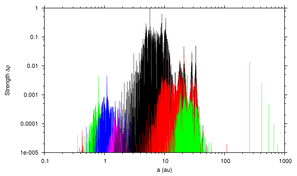## ATLAS OF THREE BODY MEAN MOTION RESONANCES IN THE SOLAR SYSTEM

Tabare Gallardo
www.fisica.edu.uy/~gallardoThe present plots, tables and programs give the location and estimated strength in relative units of the three-body resonances between a massless particle and two planets. The estimated strength Delta rho was calculated following Gallardo (2014, Icarus 231, 273-286). It depends on the eccentricity, inclination and the argument of perihelion (w) of the particle's orbit. For more details see preprint here.

### Plots

• From 0 to 1000 au. Code colors: resonances involving Mercury as the most inner planet = red, Venus=green, Earth=blue, Mars=pink, Jupiter=black, Saturn=red, Uranus = green.
• From 2 au to 3.5 au. Blue: two-body resonances, red: three-body resonances. Two-body and three-body resonances are not to the same scale.

### Use

Suppose you are studying an asteroid or comet and you want to know if it is in a resonant motion. You have two options:
• Look at the tables for the strongest resonances near the semimayor axis of the body's orbit. Then compute the corresponding critical angle
`sigma = k0*lambda_ast + k1*lambda_pla1 + k2*lambda_pla2 - (k0+k1+k2)*longper_ast `
• Or run the program ATLAS3BR and compute the strengths of all resonances near the semimajor axis of the particle according to the values of (e,i,w) of the particle's orbit. This is the best choice. Then compute and look at the time evolution of the critical angles corresponding to the strongest resonances in the interval.

### Limitations

The semimajor axis of the actual resonances can be something different from this theory because we have not taken into account the shift in "a" generated by the time evolution of the perihelion and node of the particle's orbit. For larger semimajor axes we have larger errors in "a".